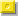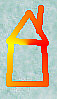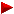HOME plateWON | World!OfNumbersNumbers whose digits occurwith same frequency ~ Classification P2Class_P3Class_P4Class_P5

Introduction

Concept originated dd.[ December 27, 1999 ]

Find more smallest Squares, Cubes and higher Powers whose digits occur a same number of times ?
E.g. 100112 = 100220121 has exactly three zero's, three ones and three two's !
Note that the number of different digits in the decimal expansion may differ from their required frequency.
The next tables already give most solutions for frequencies 1 to 6 for the powers 2 to 5.
Though the numbers are definitely 'base-related' they surely display a notable beauty !

Some of the first questions I came up with are :
Are there always solutions possible for any frequency ?
What are the possible shortcuts one can make while programming this puzzle ?

The Tables

Freq-
uency
Square Root
A052069
Square A052070
General Classification for case P2
Class
100P2(1.1)A
2887744P2(2.2)A
310.011100220121P2(3.3)A
431.646.1911001481404808481P2(4.4)A
516.431.563
3.180.566.996
269996262622969
10116006416044464016 (is next one)
P2(3.5)A
P2(4.5)A
6667.567.716445646655445456656
by Jeff Heleen - [ January 17, 2000 ]
P2(3.6)A
710.715.008.859114811414848448481881
by Jeff Heleen - [ January 20, 2000 ]
P2(3.7)A
8652.246.443.112425425422552255452244544
by Jon E. Schoenfield - [ August 18, 2007 ]
P2(3.8)A
915.647.628.653.832244848282488224248488284224
by Jon E. Schoenfield - [ August 18, 2007 ]
P2(3.9)A
10???
11???

Freq-
uency
Cube Root
A052071
Cube A052072
General Classification for case P3
Class
100P3(1.1)A
2111331P3(2.2)A
3888700227072P3(3.3)A
42.830.47922676697737363992239P3(5.4)A
5120.023.1421728999927211172788179288
by Jeff Heleen - [ January 21, 2000 ]
P3(5.5)A
66.351.783.105256263633328535368685258882625
by Jeff Heleen - [ January 25, 2000 ]
P3(5.6)A
7???
8???
9???
10???
11???

Freq-
uency
4th Root
A052093
4th Power (by Jeff Heleen) A052094
General Classification for case P4
Class
100P4(1.1)A
22071836036801P4(5.2)A
3130.398289123718973983667216P4(7.3)A
45.694.2071051315345334684056886604801P4(7.4)A
5426.424.82833065106952901022329359695121613056P4(7.5)A
6562.379.596.107100027225332362313560971766691937509957725591601P4(8.6)A
7???
8???
9???
10???
11???

Freq-
uency
5th Root
A054212
5th Power (by Jeff Heleen) A054213
General Classification for case P5
Class
100P5(1.1)A
22.955225313610074846875P5(9.2)A
349.995312343781246875156246875P5(8.3)A
410.365.589119665765800843104737370354851986949P5(9.4)A
575.418.3842439979134100773706931016420916722663424P5(8.5)A
62.592.877.410117195225794292252449115584887987847895470100000P5(8.6)A
7???
8???
9???
10???
11???

```

```ClassificationTake a look at the following table and decide if you want to partake to complete it.
The task looks formidable but with joined efforts I think we can make a success.

Frequency with which the digits occur in Pn
'd' differ.
digits
1234567...
11.11.21.31.41.51.61.7...
22.12.22.32.42.52.62.7...
33.13.23.33.43.53.63.7...
44.14.24.34.44.54.64.7...
55.15.25.35.45.55.65.7...
66.16.26.36.46.56.66.7...
77.17.27.37.47.57.67.7...
88.18.28.38.48.58.68.7...
99.19.29.39.49.59.69.7...
1010.110.210.310.410.510.610.7...

What it means is best explained with an example.
Take for instance cell 3.2 of the square table P2.
This means we want to find the smallest, the largest and the total of all squares
whose decimal expansion contains exactly 3 different digits (from the 10 available)
and these 3 digits each occur 2 times (the order is irrelevant - but leading zero's are not allowed).
Clearly the first solution for P2(3.2) is 478 which gives square 228484.
To indicate it's the smallest solution I'll add a superscript 'A' to the notation,
a 'Z' for the largest or greatest one, and a 'T' for the total number of solutions.
The final notation for the above example becomes thus
P2(3.2)A = 4782 = 228484

```

```Classification P2Frequency with which the digits occur in P2
'd' differ.
digits
1234567...
14000000...
26100000...
3138120142...
4363871102210??...
5661659925527???...
696102020700????...
71235360?????...
89724553?????...
98398442?????...
1087468372?????...
```

```

The Making of an Exhaustive List

JH = Jeff Heleen
JES = Jon E. Schoenfield

The smallest solutions

P2(1.1)A = 02 = 0
P2(1. >1)A = nihil

P2(2.1)A = 42 = 16
P2(2.2)A = 882 = 7744 = P2(2.2)Z
P2(2. >2)A = nonexistant ?

P2(3.1)A = 132 = 169
P2(3.2)A = 4782 = 228484
P2(3.3)A = 100112 = 100220121
P2(3.4)A = nihil
P2(3.5)A = 164315632 = 269996262622969 = P2(3.5)Z
P2(3.6)A = 6675677162 = 445646655445456656
P2(3.7)A = 107150088592 = 114811414848448481881
P2(3.8)A = 6522464431122 = 425425422552255452244544 (JES)
P2(3.9)A = 156476286538322 = 244848282488224248488284224 (JES)

P2(4.1)A = 322 = 1024
P2(4.2)A = 33622 = 11303044
P2(4.3)A = 3333032 = 111090889809
P2(4.4)A = 316461912 = 1001481404808481
P2(4.5)A = 31805669962 = 10116006416044464016
P2(4.6)A = 3173149865462 = 100688800686688161010116
P2(4.7)A = 316940238122902 = 1004511145414005545155044100

P2(5.1)A = 1132 = 12769
P2(5.2)A = 321512 = 1033686801
P2(5.3)A = 100064222 = 100128481242084
P2(5.4)A = 31624321612 = 10000977172927129921
P2(5.5)A = 10000249591962 = 1000049919014961464966416

P2(6.1)A = 3222 = 103684
P2(6.2)A = 3165282 = 100189974784
P2(6.3)A = 3163276772 = 100063199236216329
P2(6.4)A = 3162298952212 = 100001346631484638638841
P2(6.5)A = 3162279837363192 = 100000137697937633971319669761

P2(7.1)A = 10172 = 1034289
P2(7.2)A = 31659592 = 10023296389681
P2(7.3)A = 100005868562 = 100011737464399964736

P2(8.1)A = 32062 = 10278436
P2(8.2)A = 316452582 = 1001422353886564
P2(8.3)A = 3162457231142 = 100011357387896753856996

P2(9.1)A = 101242 = 102495376
P2(9.2)A = 3164246172 = 100124538243596689
P2(9.3)A = 100005622141352 = 100011244598784733593798225

P2(10.1)A = 320432 = 1026753849
P2(10.2)A = 31642527362 = 10012495377283485696
P2(10.3)A = 3162455099884262 = 100011222587839648934653957476

The largest solutions

P2(1.1)Z = 32 = 9
P2(1. >1)Z = nihil

P2(2.1)Z = 92 = 81
P2(2.2)Z = 882 = 7744 = P2(2.2)A
P2(2. >2)Z = nihil ?

P2(3.1)Z = 312 = 961
P2(3.2)Z = 8932 = 797449
P2(3.3)Z = 310862 = 966339396
P2(3.4)Z = nihil
P2(3.5)Z = 164315632 = 269996262622969 = P2(3.5)A
P2(3.6)Z = 8954391002 = 801811181808810000
P2(3.7)Z = 150085150002 = 225255522505225000000

P2(4.1)Z = 992 = 9801
P2(4.2)Z = 99332 = 98664489
P2(4.3)Z = 9997022 = 999404088804
P2(4.4)Z = 999933332 = 9998666644448889
P2(4.5)Z = 99999333332 = 99998666664444488889
P2(4.6)Z = 9999993333332 = 999998666666444444888889
P2(4.7)Z = 999999933333332 = 9999998666666644444448888889

P2(5.1)Z = 3112 = 96721
P2(5.2)Z = 993302 = 9866448900
P2(5.3)Z = 316204502 = 999852858202500
P2(5.4)Z = 99999415722 = 99998831443413831184
P2(5.5)Z = 31622131674852 = 9999592116615516661225225 (JH)
P2(5.6)Z = 9999993644859392 = 999998728972281878121728711721 (JH)

P2(6.1)Z = 9682 = 937024
P2(6.2)Z = 9975352 = 995076076225
P2(6.3)Z = 9999377492 = 999875501875187001
P2(6.4)Z = 9999941572002 = 999988314434138311840000
P2(6.5)Z = 9999994032782262 = 999998806556808076875565707076 (JH)

P2(7.1)Z = 31422 = 9872164
P2(7.2)Z = 99943022 = 99886072467204
P2(7.3)Z = 316209872042 = 999886831755531737616

P2(8.1)Z = 99162 = 98327056
P2(8.2)Z = 999377892 = 9987561670208521
P2(8.3)Z = 9999443616812 = 999888726457622541145761

P2(9.1)Z = 303842 = 923187456
P2(9.2)Z = 9994166812 = 998833702261055761
P2(9.3)Z = 316210178081822 = 999888767225363175346145124

P2(10.1)Z = 990662 = 9814072356
P2(10.2)Z = 99943634882 = 99887301530267526144
P2(10.3)Z = 9999443871187112 = 999888777330214565264406301521

The total number of solutions

P2(1.1)T = 4

02 = 0
12 = 1
22 = 4
32 = 9
P2(1. >1)T = 0From Charles W. Trigg's book "Mathematical Quickies", Dover Publications 1985, Q27 page 10 and 88.
I quote the reasoning that there exist no squares composed of only identical digits.

1. A square can end only in 0, 1, 4, 5, 6 or 9.
2. The tens' digit of a square number is even unless the units' digit is 6,
in which event it is odd.
Thus the digits cannot all be the same unless they are all 4's. (A square
consisting only of zeros is obviously meaningless.) But ...444 = 4(...111)
and since the tens' digit inside the parentheses is odd, no square integer
in the decimal system can consist of digits all alike.

P2(2.1)T = 6
42 = 16
52 = 25
62 = 36
72 = 49
82 = 64
92 = 81
P2(2.2)T = 1    a unique solution !
882 = 7744
P2(2. >2)T = 0    all nonexistant ?The following source states that squares using only two distinct digits, not ending in 0,
are probably finite (no other term < 10^41) - A016069 and A018884.
The largest known such square is 816192 = 6661661161.

P2(3.1)T = 13
132 = 169  |  182 = 324  |  252 = 625  |  312 = 961
142 = 196  |  192 = 361  |  272 = 729
162 = 256  |  232 = 529  |  282 = 784
172 = 289  |  242 = 576  |  292 = 841
P2(3.2)T = 8
4782 = 228484  |  8362 = 698896
5772 = 332929  |  8802 = 774400
5832 = 339889  |  8812 = 776161
7152 = 511225  |  8932 = 797449
P2(3.3)T = 12
100112 = 100220121  |  110102 = 121220100  |  220022 = 484088004
101102 = 102212100  |  144992 = 210221001  |  220202 = 484880400
104012 = 108180801  |  200222 = 400880484  |  289982 = 840884004
110012 = 121022001  |  202202 = 408848400  |  310862 = 966339396
P2(3.4)T = 0
P2(3.5)T = 1    a unique Prime Curios! solution !
164315632 = 269996262622969
P2(3.6)T = 4
6675677162 = 445646655445456656
6960376922 = 484468468684686864
8178519542 = 668881818661618116
8954391002 = 801811181808810000
P2(3.7)T = 2
107150088592 = 114811414848448481881
150085150002 = 225255522505225000000

P2(4.1)T = 36
P2(4.2)T = 38
P2(4.3)T = 71
P2(4.4)T = 102
P2(4.5)T = 210 (JH)

P2(5.1)T = 66
P2(5.2)T = 165
P2(5.3)T = 992
P2(5.4)T = 5527 (JH)
P2(5.5)T = ?

P2(6.1)T = 96
P2(6.2)T = 1020
P2(6.3)T = 20700 (JH)

P2(7.1)T = 123
P2(7.2)T = 5360
P2(7.3)T = ?

P2(8.1)T = 97
P2(8.2)T = 24553
P2(8.3)T = ?

P2(9.1)T = 83
P2(9.2)T = 98442 (JH)

P2(10.1)T = 87
P2(10.2)T = 468372 (JH)

While working on the data for this page I came up with the following
infinite pattern that I like to share with you :

932 = 8649
99332 = 98664489
9993332 = 998666444889
999933332 = 9998666644448889
99999333332 = 99998666664444488889

Each square belongs to the general classification P2(4.n) with n = 1, 2, 3, 4, 5, etc.

This one was sent in by Jeff Heleen where he noticed that the root consists of only 2 digits

3333032 = 111090889809
333330032 = 1111089088998009
33333300032 = 11111088908899980009

Each square belongs to the general classification P2(4.n) with n = 3, 4, 5, etc.

332 = 1089 could belong to the pattern also if the condition of the root having 2 distinct digits is dropped.
But since I haven't a solution for P2(4.2) of this kind, the pattern's smoothness is lost!

Another neat arrangement of digits is for this member of P2(4.5):
36001800362 = 1296.1296.2916.1296.1296
Periods used here only to separate out the interesting stuff.

Here is my collection of such numbers with palindromic squareroots.
02 = 0
12 = 1
22 = 4
32 = 9
42 = 16
52 = 25
62 = 36
72 = 49
82 = 64
92 = 81
332 = 1089
442 = 1936
552 = 3025
662 = 4356
882 = 7744
992 = 9801
1812 = 32761
1912 = 36481
2322 = 53824
2522 = 63504
2722 = 73984
2822 = 79524
2922 = 85264
3232 = 104329
3532 = 124609
6162 = 379456
6262 = 391876
6862 = 470596
7172 = 514089
7372 = 543169
7572 = 573049
7772 = 603729
7972 = 635209
9292 = 863041
14412 = 2076481
19912 = 3964081
25522 = 6512704
28822 = 8305924
29922 = 8952064
45542 = 20738916
75572 = 57108249
77772 = 60481729
104012 = 108180801
285822 = 816930724
324232 = 1051250929
358532 = 1285437609    pandigital
401042 = 1608330816
504052 = 2540664025
507052 = 2570997025
846482 = 7165283904    pandigital
977792 = 9560732841    pandigital

Contributions

Jeff Heleen (email) from New Hampshire, USA.

```

```

[TOP OF PAGE]

Patrick De Geest - Belgium- Short Bio - Some Pictures